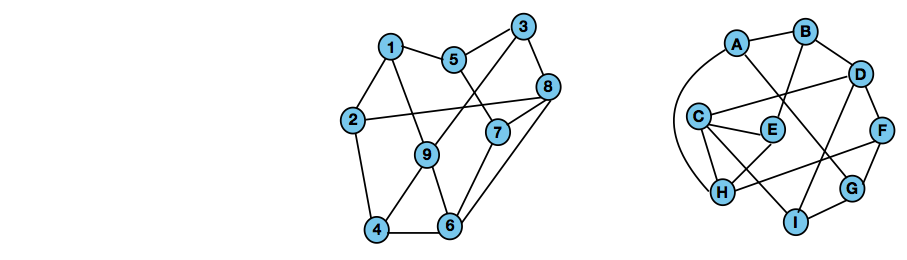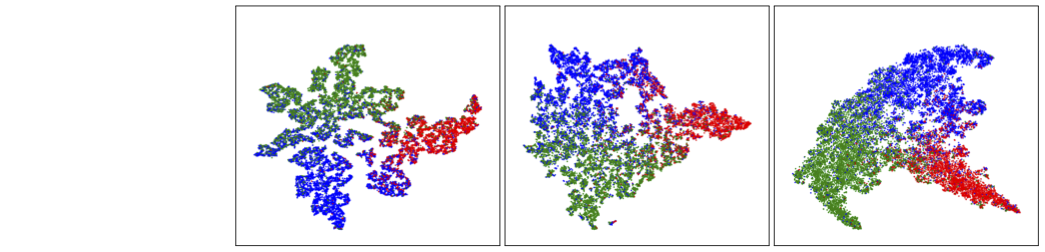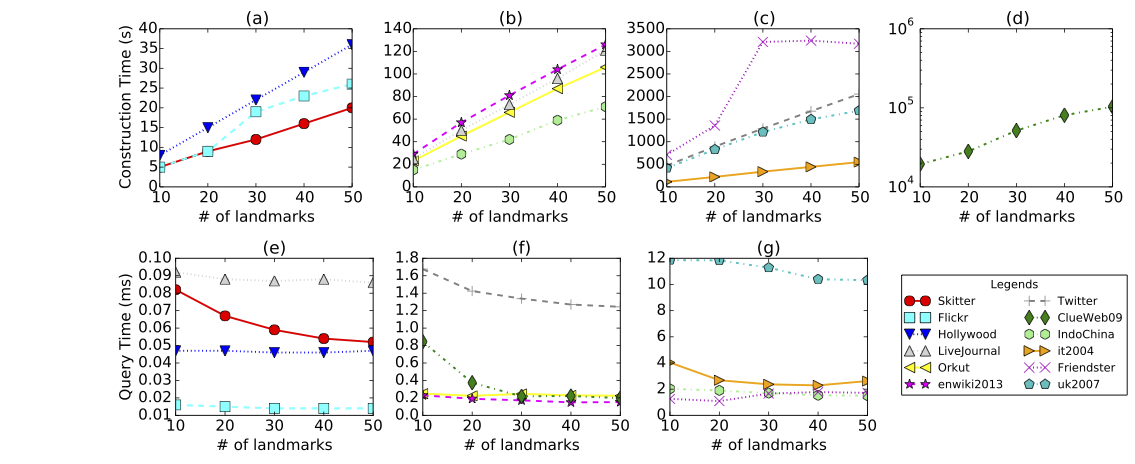### Current Projects

Below are some projects that we currently work on:

Deep Learning for Graph Isomorphism. Graph isomorphism is a fundamental concept for exploiting the structure of graphs. This project aims to develop new heuristic techniques and theories for graph isomorphism, advancing state-of-the-art methodologies for its applications in solving real-world problems. Inspired by recent advances in machine learning, this project will investigate graph isomorphism problems from a deep learning perspective by marrying the best approaches from classic graph isomorphism studies with new techniques from modern AI. This is an important step towards bridging the gap between combinatorical generalization and deep learning.

Below is an example of an isomorphism between two graphs, which is the mapping: 1 → A, 2 → B, 3 → F, 4 → E, 5 → G, 6 → C, 7 → I, 8 → D, 9 → H (practical problems can have millions of vertices):Geometric Graph Learning. Geometric deep learning is an emerging field of machine learning, which aims to apply deep learning techniques to learn from complex data like graphs and multi-dimensional points. In recent years, due to the rising trends in network analysis and prediction, Graph Neural Networks (GNNs) as a powerful deep learning approach have been widely applied in various fields, e.g., object recognition, image classification, and semantic segmentation. However, graphs are in irregular non-Euclidean domains. This brings up the challenge of how to design deep learning techniques in order to effectively extract useful features from arbitrary graphs. In this project, we aim to explore the mathematical foundations of GNNs and develop a general framework for deriving neural network architectures on graphs in a principled way.

Below is the t-SNE visualization of the 2-D node embedding space for the Pubmed dataset by applying our Distributed Feedback-Looped Networks (DFNets):Shortest-Path Algorithms. Finding the shortest-path information (e.g., distance, paths, counting of paths) between a pair of vertices is a fundamental task in graph theory, and has a broad range of applications. For example, in web graphs, ranking of web pages based on their distances to recently visited web pages helps in finding the more relevant pages and is referred to as context-aware search. In social network analysis, distance is used as a core measure in many problems such as centrality and community identification. However, despite extensive efforts in addressing the shortest-path problems for many years, there is still a high demand for scalable solutions that can be used to support analysis tasks over large and ever-growing networks. Questions of interest include: (i), How to develop robust, efficient and scalable solutions for shortest-path related problems on large networks with possibly billions of vertices? (ii) How to handle dynamics of networks, i.e., topological structure changes caused by node and edge insertions and deletions? (iii), What kinds of the theoretical properties can we gurantee in our soltions?

The figure below shows that our Highway Labelling (HL) technique can construct distance labelling linearly in terms of the number of landmarks while answering distance queries on large networks efficiently (on the scale of billions).